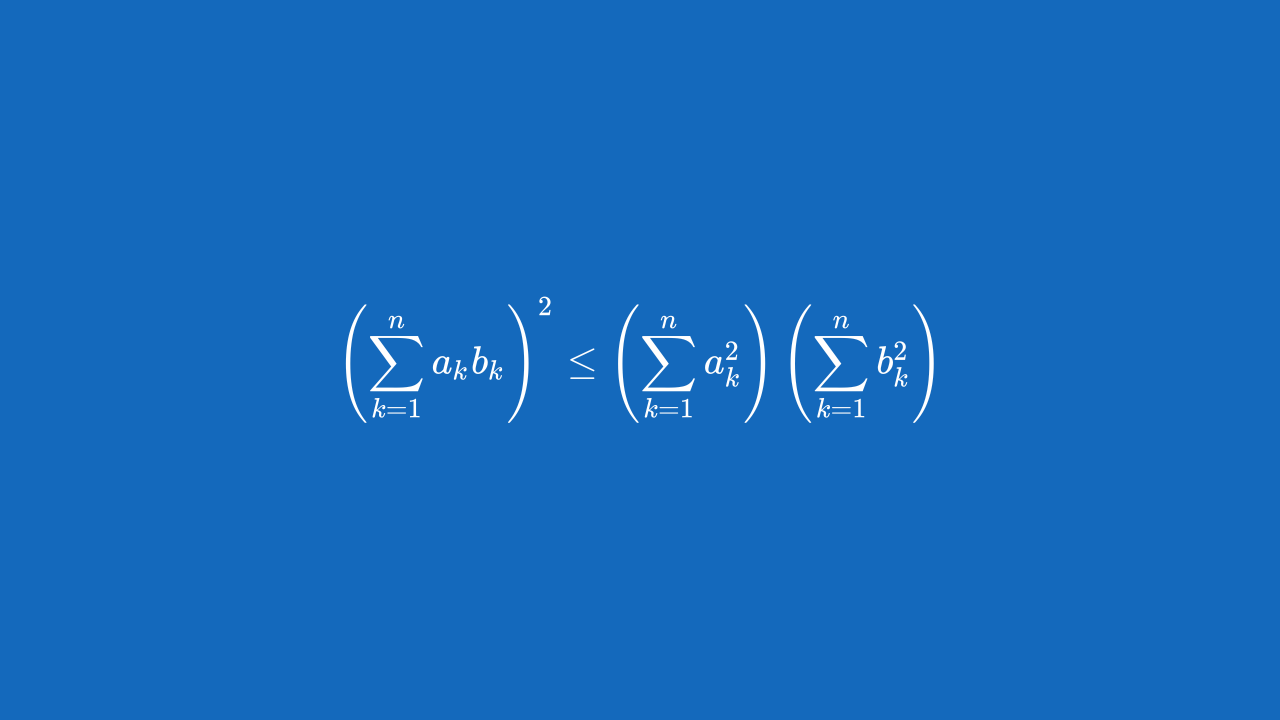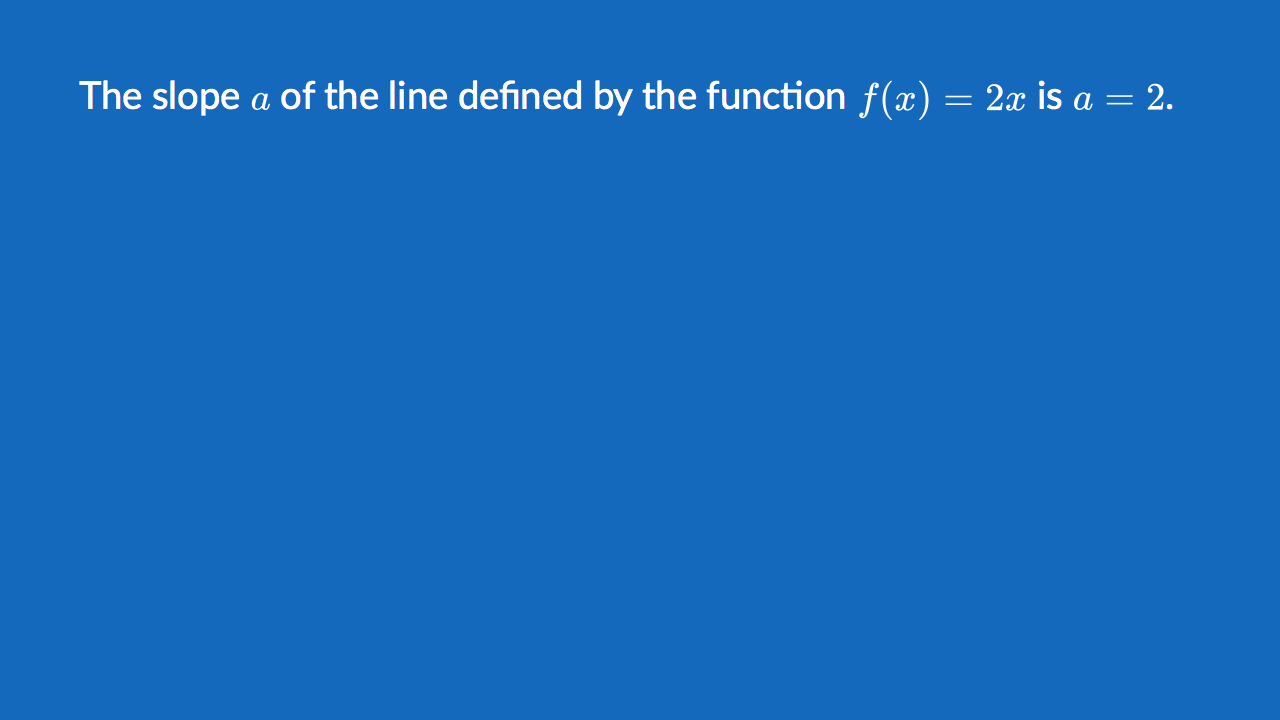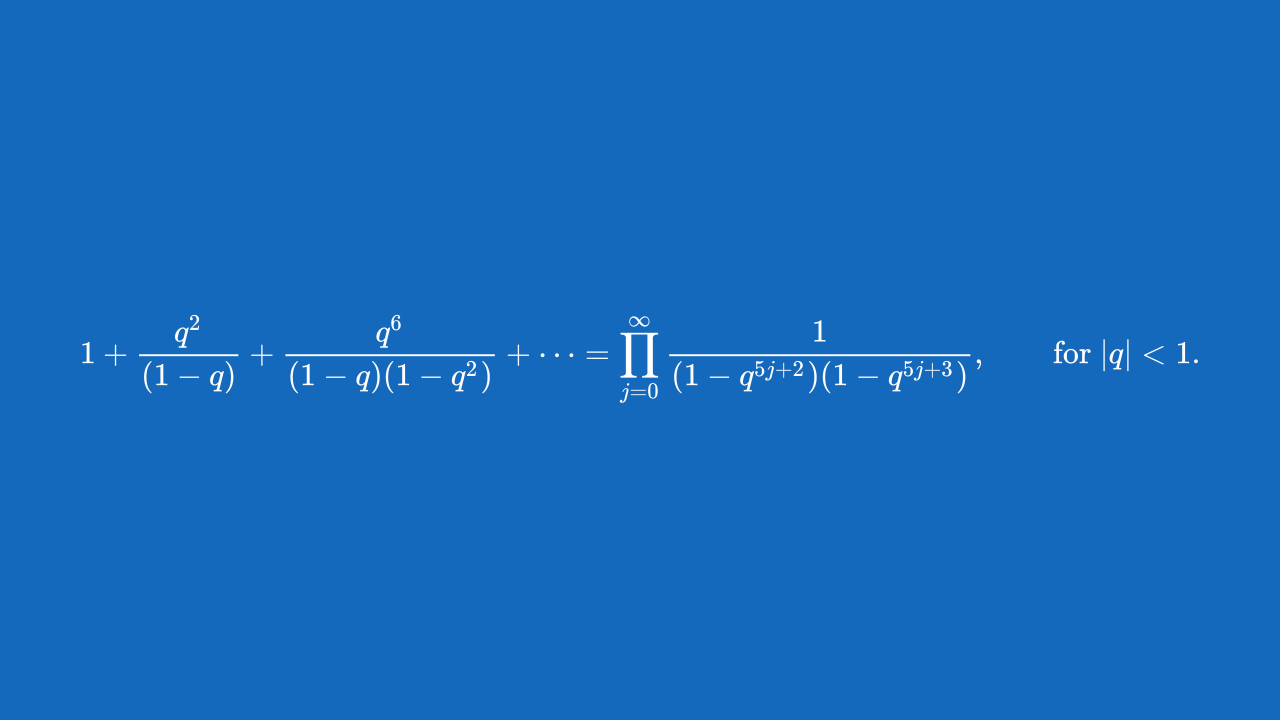# Formulas

Easily include mathematical formulas by enclosing TeX commands in $$ delimiters. Deckset uses MathJax to translate TeX commands into beautiful vector graphics. $$
\left( \sum_{k=1}^n a_k b_k \right)^2 \leq \left( \sum_{k=1}^n a_k^2 \right) \left( \sum_{k=1}^n b_k^2 \right)
$$## Inline Formulas You can also include Formulas in paragraph text. Deckset takes care of adjusting the font size and color to match the surrounding text, for example: The slope$$a$$of the line defined by the function$$f(x) = 2x$$is$$a = 2$$.## Formula Autoscaling Don’t worry if your equations get really complex. Deckset will scale them down to fit onto the slide. $$
1 +  \frac{q^2}{(1-q)}+\frac{q^6}{(1-q)(1-q^2)}+\cdots =
\prod_{j=0}^{\infty}\frac{1}{(1-q^{5j+2})(1-q^{5j+3})},
\quad\quad \text{for $|q|<1$}.